• ## 向量投影

万次阅读 2018-04-18 15:36:49
向量投影给定一个向量u和v，求u在v上的投影向量，如下图。假设u在v上的投影向量是u’，且向量u和v的夹角为theta。一个向量有两个属性，大小和方向，我们先确定u’的大小（即长度，或者模），从u的末端做v的垂线，...
向量投影给定一个向量u和v，求u在v上的投影向量，如下图。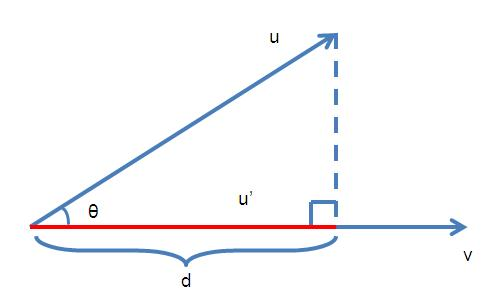假设u在v上的投影向量是u’，且向量u和v的夹角为theta。一个向量有两个属性，大小和方向，我们先确定u’的大小（即长度，或者模），从u的末端做v的垂线，那么d就是u’的长度。而u’和v的方向是相同的，v的方向v/|v|也就是u’的方向。所以有(1)再求d的长度。(2)最后求cos(theta)(3)联合求解方程（1）（2）（3）得到这就是最终的投影向量。而这个向量的长度d是============================以下是旧的推导，也保留。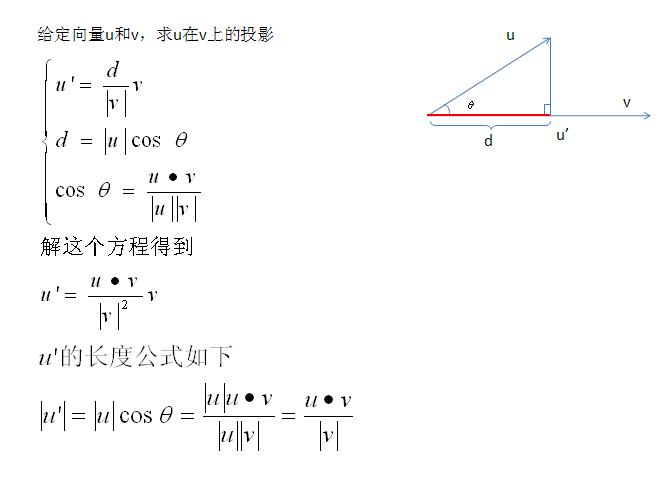展开全文• 向量投影向量投影矩阵 向量投影 以下是向量a在向量b上的投影，θ 为两向量的夹角。 其中a = a||+a⊥，a||则是a在b上的投影。 所以投影公式如下： 向量投影矩阵 将以上投影公式写成矩阵形式，...
向量投影与向量投影矩阵

向量投影

以下是向量a在向量b上的投影，θ 为两向量的夹角。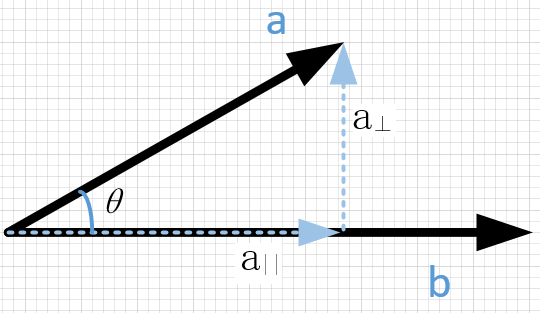其中a = a||+a⊥，a||则是a在b上的投影。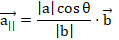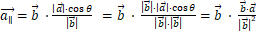所以投影公式如下：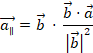向量投影矩阵

将以上投影公式写成矩阵形式，这里使用的是列优先的矩阵，即向量写成一列多行。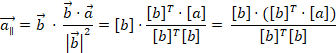因为矩阵相乘符和结合律，所以：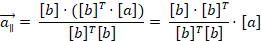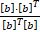是一个矩阵，如果是二维向量则是2*2的矩阵，如果是三维向量则是一个3*3的矩阵，这个也就是向量投影矩阵。

将向量投影矩阵记作P，则：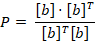P矩阵有些特性，首先P矩阵是一个对称矩阵，所以转置矩阵PT = P。其次如果一个向量在另一个向量上进行多次投影，其结果相等的，所以P^2 = P

也就有 PT = P = P^2 = P^3 = … = P^n

参考：

https://blog.csdn.net/williamgavin/article/details/77427164

《3D数学基础：图形与游戏开发》—— 5.10.3

展开全文• 向量投影 力的正交分解就是投影，高中时一般向坐标轴投影，有时也需计算力在任意方向分量，即力在这个方向的投影，可通过内积计算。但有时需要计算力在某个平面内的分量，即力在平面内的投影，或者计算力垂直于某...
向量投影
力的正交分解就是投影，高中时一般向坐标轴投影，有时也需计算力在任意方向分量，即力在这个方向的投影，可通过内积计算。但有时需要计算力在某个平面内的分量，即力在平面内的投影，或者计算力垂直于某平面的分量，都可通过投影解决。
几何上，也经常涉及投影，如点到直线或平面的距离，此距离是点到直线或平面的最短距离，求此距离可通过投影解决。
再举个例子，为什么称为“投影”，投影就是物体在太阳光的照射下在地面形成的影子。当太阳光与地面垂直时是正投影，这就是线性代数中研究的投影。当物体与地面垂直时，影子长度（投影）为0。中国古时利用投影来计时，发明了日晷。希望读者根据物理学和几何学，获得投影的几何图像。
当转向高维空间时，投影不局限于低维子空间，可投向任意维度的子空间，所以需要代数方法，但理解需要几何图像。向量在子空间的投影，本质上是向量的正交分解，分解为两个向量：一个向量位于子空间，另一个位于其正交补空间。
子空间 $S_1$ 由无关组 $V = (\mathbf{v_1},\cdots,\mathbf{v_n})$ 张成，如何求任意向量 $\mathbf{v}$ 在子空间的投影向量呢？这是线性代数基本问题之一，也是理解线性方程的核心之一，必须十分重视。
投影向量位于子空间 $S_1$ 内，故其可表示为生成向量的线性组合，这是解决问题的关键一步，令投影向量为 $\mathbf{v}^{\bot} = \alpha_1\mathbf{v_1}+\cdots+\alpha_n\mathbf{v_n}$ ，只要算出表示系数组就可得到投影向量。另一正交分量 $\mathbf{v}^{-} = \mathbf{v}-\mathbf{v}^{\bot}$ 位于正交补空间 $S_1^{\bot}$ ，垂直子空间 $S_1$ 内任意向量。故两个分向量垂直，内积为零！这是关键第二步。
$0 = (\mathbf{v}^{\bot}, \mathbf{v}^{-}) = (\mathbf{v}^{\bot}, \mathbf{v}-\mathbf{v}^{\bot}) = (\mathbf{v}^{\bot}, \mathbf{v}) - (\mathbf{v}^{\bot}, \mathbf{v}^{\bot})\\ 得：(\mathbf{v}^{\bot}, \mathbf{v}) = (\mathbf{v}^{\bot}, \mathbf{v}^{\bot}) \\ 得：(\alpha_1\mathbf{v_1}+\cdots+\alpha_n\mathbf{v_n}, \mathbf{v}) =(\alpha_1\mathbf{v_1}+\cdots+\alpha_n\mathbf{v_n},\alpha_1\mathbf{v_1}+\cdots+\alpha_n\mathbf{v_n})=\sum_{ij}\alpha_i\alpha_j(\mathbf{v_i},\mathbf{v_j})$
根据正交分解的唯一性，$\mathbf{v}^{\bot}$ 是唯一的，根据无关组表示向量的唯一性，表示系数组存在且唯一。无关组是任意向量时，利用向量理论很难表示出表示系数，必须用矩阵理论才能方便表示。
无关组是标准正交基时，却很容易求出表示系数。 根据标准正交基性质： $(\mathbf{v_i},\mathbf{v_j})=0,\forall i\ne j; \quad =1 ，\forall i= j$ 。代入上式，
$(\alpha_1\mathbf{v_1}+\cdots+\alpha_n\mathbf{v_n}, \mathbf{v}) = \alpha_1(\mathbf{v_1}, \mathbf{v})+\cdots+\alpha_n(\mathbf{v_n}, \mathbf{v}) = \alpha_1^2+\cdots+\alpha_n^2$
显然当 $\alpha_i = (\mathbf{v_i}, \mathbf{v}), \forall i \in [1,n]$ 时，等式恒成立！故投影向量为：
$\mathbf{v}^{\bot}=(\mathbf{v_1}, \mathbf{v})\mathbf{v_1}+\cdots+(\mathbf{v_n}, \mathbf{v})\mathbf{v_n}$
标准正交基可以看作坐标轴，向量与坐标轴的内积就是向量在该轴方向的分量（坐标值），子空间内所有坐标轴方向的分量相加就是投影！
特别重要的特例，当子空间就是整个空间时，显然投影向量就是向量本身，得：
重要性质 $m$ 维空间任意向量 $\mathbf{v}$ 与标准正交基 $V$ 的正交分解：
$\mathbf{v}=(\mathbf{v_1}, \mathbf{v})\mathbf{v_1}+\cdots+(\mathbf{v_m}, \mathbf{v})\mathbf{v_m}$
这就是物理学中的正交分解！得到最简基节同样结论。
下面证明垂线距离最短，几何上就是直角三角形斜边长度大于直角边，线性代数也是这样证明的。假设子空间 $S_1$ 内任意向量 $\mathbf{u}$ ，向量 $\mathbf{v}$ 分解为 $\mathbf{v}=\mathbf{u}+\mathbf{w}$ ，则向量 $\mathbf{w}$ 是斜边，向量 $\mathbf{v}-\mathbf{v}^\bot$ 是垂线，请想象出二维点到直线距离，三维点到平面距离的图像。
$\|\mathbf{w}\|^2 = \|\mathbf{v}-\mathbf{u}\|^2=\|(\mathbf{v}-\mathbf{v}^\bot) + (\mathbf{v}^\bot-\mathbf{u})\|^2 = \|(\mathbf{v}-\mathbf{v}^\bot)\|^2 + \|(\mathbf{v}^\bot-\mathbf{u})\|^2 \ge \|(\mathbf{v}-\mathbf{v}^\bot)\|^2$
向量 $\mathbf{v}-\mathbf{v}^{\bot}$ 位于正交补空间 $S_1^{\bot}$ ，垂直子空间内任意向量，向量 $(\mathbf{v}^\bot,\mathbf{u})$  位于子空间内，故 $\mathbf{v}-\mathbf{v}^{\bot}$ 垂直 $(\mathbf{v}^\bot-\mathbf{u})$ ，根据勾股定理，得到中间等式。
向量投影有个应用，就是判断向量是否位于子空间内？如果向量等于子空间投影，则向量位于子空间内。子空间内的向量能被子空间的生成向量组表示。
向量投影的这两个性质对于理解线性方程很关键，特别是最小二乘法。


展开全文线性代数 矩阵
• ## 向量投影的性质

千次阅读 2018-03-27 21:53:57
向量aaa在向量uuu上的投影记为 Prju→a→Prju→a→...向量投影的性质： Prju→a→=|a→|cosϕPrju→a→=|a→|cos⁡ϕPrj_\overrightarrow u \overrightarrow a = |\overrightarrow a|\cos \phi，其中ϕϕ\phi为a...
向量aa<!--//--><![CDATA[//><!--
a
//--><!]]>在向量uu<!--//--><![CDATA[//><!--
u
//--><!]]>上的投影记为 Prju→a→Prju→a→<!--//--><![CDATA[//><!--
Prj_{\overrightarrow u} \overrightarrow a
//--><!]]>

向量投影的性质：

Prju→a→=|a→|cosϕPrju→a→=|a→|cos⁡ϕ<!--//--><![CDATA[//><!--
Prj_\overrightarrow u \overrightarrow a = |\overrightarrow a|\cos \phi
//--><!]]>，其中ϕϕ<!--//--><![CDATA[//><!--
\phi
//--><!]]>为a→a→<!--//--><![CDATA[//><!--
\overrightarrow a
//--><!]]>与u→u→<!--//--><![CDATA[//><!--
\overrightarrow u
//--><!]]>的夹角
Prju→(a→+b→)=Prju→a→+Prju→b→Prju→(a→+b→)=Prju→a→+Prju→b→<!--//--><![CDATA[//><!--
Prj_\overrightarrow u (\overrightarrow a + \overrightarrow b) = Prj_\overrightarrow u \overrightarrow a + Prj_\overrightarrow u \overrightarrow b
//--><!]]>
Prju→λa→=λPrju→a→Prju→λa→=λPrju→a→<!--//--><![CDATA[//><!--
Prj_\overrightarrow u \lambda \overrightarrow a = \lambda Prj_\overrightarrow u \overrightarrow a
//--><!]]>
对于第一条性质，在计算时常常可以化简： cosϕ=a→⋅b→|a→||b→|cos⁡ϕ=a→·b→|a→||b→|<!--//--><![CDATA[//><!--
\cos \phi = \frac{\overrightarrow a · \overrightarrow b}{|\overrightarrow a| |\overrightarrow b|}
//--><!]]>⇒Prju→a→=|a→|cosϕ=|a→|a→⋅b→|a→||b→|⇒Prju→a→=|a→|cos⁡ϕ=|a→|a→·b→|a→||b→|<!--//--><![CDATA[//><!--
\Rightarrow Prj_\overrightarrow u \overrightarrow a = |\overrightarrow a|\cos \phi = |\overrightarrow a|\frac{\overrightarrow a · \overrightarrow b}{|\overrightarrow a| |\overrightarrow b|}
//--><!]]>⇒Prju→a→=a→⋅b→|b→|⇒Prju→a→=a→·b→|b→|<!--//--><![CDATA[//><!--
\Rightarrow Prj_\overrightarrow u \overrightarrow a = \frac{\overrightarrow a · \overrightarrow b}{|\overrightarrow b|}
//--><!]]>
展开全文高等数学
• 点在直线的投影坐标 n维向量投影坐标 几何投影坐标 一、点在直线的投影坐标  如下图所示，直线l1：y=kx+b，直线外有一点P(x0, y0)，问：点P在直线上的投影坐标为多少呢？   求点P的投影坐标，即是求过点P(x0,...坐标变换 投影坐标
• 向量投影 如上图，求向量P在向量Q上的投影，计算方法如下： 将向量P当做直角三角形的斜边，那么投影大小就是 直接求比较麻烦，据公式可得： 则： 即投影大小等于： 投影方向与向量Q平行，求向量Q的单位矢量...计算机图形学
• 文章目录向量投影和最小二乘法1. 向量的投影1.1 向量在向量上的投影1.2 向量在空间上的投影2. 最小二乘法2.1 最小二乘法近似2.2 直线和抛物线的拟合3. 参考资料 向量投影和最小二乘法 1. 向量的投影 问题1: 某个...线性代数 最小二乘法
• https://blog.csdn.net/wlk1229/article/details/84779370
• 向量投影是线性代数中很重要的应用，用于找到向量到目标投影空间的投影向量。这是下一节线性回归的基础。 Ax=bAx=bAx=b有解时 当计算线性方程组Ax=bAx=bAx=b 有解时， bbb就在C(A)C(A)C(A)的子空间中，则Ax=bAx=...线性代数
• ## 向量投影推导

千次阅读 2020-07-13 15:07:13
给定一个向量u和v，求u在v上的投影向量，如下图。 假设u在v上的投影向量是u’，且向量u和v的夹角为theta。一个向量有两个属性，大小和方向，我们先确定u’的大小（即长度，或者模），从u的末端做v的垂线，那么d...
• ## 向量投影证明

千次阅读 2019-06-04 19:43:06
• ## 向量投影公式

千次阅读 2015-07-17 14:37:00
公式一：a.b = |a||b|cos(r) cos(r) = a.b/|a|/|b| 公式二：|c| = |a|cos(r) 公式三：|c| = a.b/|b| 公式四：c = b/|b| |c| 公式五：c = a.b/|b|2 b 公式六：c = a.b/b.b b...朝向解P1-P2 = 向量ARotationFromX...
• 已知向量a,b求向量b在向量a上的投影 1 b的投影向量=Vector3.Dot(a.normalized,b)*a.normalized; 2 b的投影向量=(Vector3.Dot(a,b)/a.magnitude)*a.normalized; 3 将向量b分解为垂直于向量a和平行于向量b的两个...游戏开发
• 这篇文章来自李青等的论文《基于向量投影的支持向量机预选取》。  基本思想是，m1和m2分别是1类样本，2类样本的中心点，xf(0)为样本1的一个样本，xf为xf(0)投影到m1m2的点。分类样本的中心点很好
• ## 向量投影的计算

千次阅读 2019-05-31 15:12:59
• 官方例图 测试： code: public Transform point1; public Transform point2; public Transform humanPoint; public Transform targetPoint; void Start() { } void Update() ... targe...
• 首先来看一个向量在另一个向量上的投影： 若v向量为单位向量,则结果为 uv向量的点积 * v向量. 即: 某个方向力u, 在v方向上的分量. 假如V向量我们定义为单位向量，那么U向量在V向量上的投影向量...
• 4个子空间： 行空间，0... 什么是正交向量？两个向量夹角是90度。 直角意味着：x + y 所得到的新向量 = x + y |x|^2 + |y|^2 = |x+y|^2 怎么证明呢？ x’*x + y’*y = (x+y)’*(x+y) = (x’+y’)*(x+y) =x*x’ + y...
• # coding=utf-8 from math import sqrt, acos, pi class Vector(object): ...根据坐标轴列表输入 创建向量, 并创建该向量所处的空间维度""" CANNOT_NORMALIZE_ZERO_VECTOR_MSG = 'Cannot normali
• 我们将向量 aaa作为这个直线的基向量向量 ppp就可以用 aaa来表示，记作： p=x^ap=\hat x ap=x^a（ x^\hat xx^是一个标量），我们的目标就是求取 x^\hat xx^，投影向量 ppp和投影矩阵 PPP。 我们仍然把握一个核心，...机器学习 算法 人工智能 python 线性代数
• //向量在另外两个向量的片面投影和这个面上一个向量的夹角 private float AngleCommon(Vector3 move, Vector3 to, Vector3 side_1, Vector3 side_2) { Vector3 nor = Vector3.Cross(side_1, side_2).normalized; ......